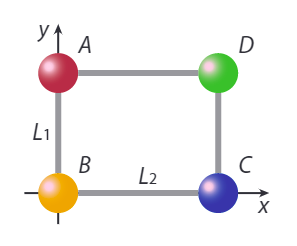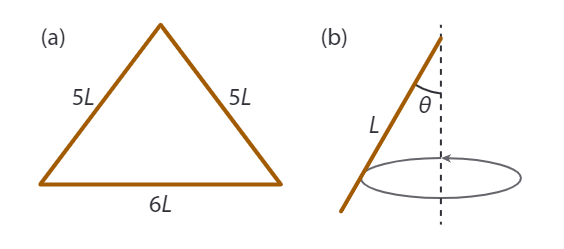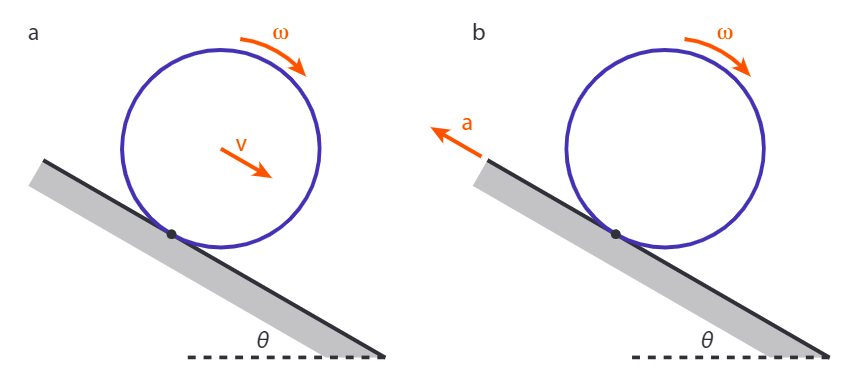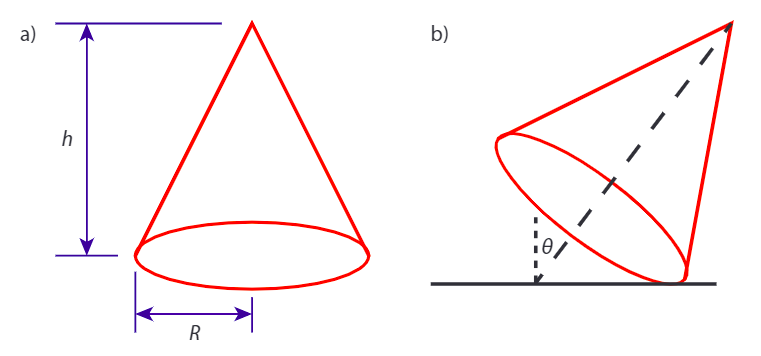$$\require{cancel}$$

5.E: Rotational Motion, Torque and Angular Momentum (Exercises)

5.1 Figure 5.E.1 shows two hand powered drills.

1.  With which of the two drills shown in Figure 5.E.1 will you be able to exert a greater torque on the drill bit?
2.  With which of the two drills shown in Figure 5.E.1 will you be able to exert a greater rotational speed?Figure $$\PageIndex{1}$$: Two hand-powered drills. (a) A brace , CC BY-SA 3.0. (b) An egg-beater drill .

5.2  An Atwood’s machine consists of two masses $$m_1$$ and $$m_2$$, connected by a string that passes over a pulley. If the pulley is a disk of radius R and mass M, find the acceleration of the masses.

5.3  You hold a uniform pen with a mass of 25.0 g horizontal with your thumb pushing down on one end and your index finger pushing upward 2.0 cm from your thumb. The pen is 14 cm long.

1.   Which of your fingers exerts the greater force?
2.   Find the two forces.

5.4  A wagon wheel is constructed as shown in the figure below.  The radius of the wheel is R.  Each of the spokes that lie along the diameter has a mass m, and the rim has mass M (you may assume the thickness of the rim and spokes are negligible compared to the radius R).1.   What is the moment of inertia of the wheel about an axis through the center, perpendicular to theplane of the wheel (figure a)?
2.   For  the  same  wheel,  what  is  the  moment  of  inertia  for  an  axis  through  the  center  and  two  of  the spokes, in the plane of the wheel (figure b)?

5.5  A sphere with radius R=0.200 m has a density $$\rho$$ that decreases with distance r from the center of the sphere, according to $$\rho(r)=a-b r$$, where $$a=1.00 \cdot 103 kg/m^3$$ and $$b=4.00 \cdot 103 kg/m^4$$.

(a)  Calculate the total mass of the sphere.

(b)  Calculate the moment of inertia of the sphere for an axis through its center.

5.6  A 0.10 kg yo-yo has an outer radius R that is 5.0 times greater than the radius r of its axle.  The yo-yo is in equilibrium if a mass m is suspended from its outer edge, as shown in Figure 5.E.1. Find the tensions $$T_1$$ and $$T_2$$ in the two strings, and the mass m.Figure $$\PageIndex{1}$$: A yo-yo with a mass suspended from its outer edge.

5.7  A table consists of three pieces:  a tabletop which is a circular disk of radius R, thickness d=R/50, and mass M; a single leg that supports the tabletop in its center and consists of a hollow cylinder of height h=R/2 and mass m=M/5,  and a foot,  which consists of a solid cylinder of radius R/3,  mass M and height h=R/4.

1.   Find the position of the center of mass of this table.
2.   With what force should you push down on the edge of the table to make it tip over?
3.   A stone of mass m=M/2 is placed on the table.  How far out from the center can it be positioned before the table tips over? You may approximate the stone as a point mass.

5.8  A set-square protractor made of plastic with density $$\rho = 1.2 g/cm^3$$, thickness 2.0 mm and sides of 10 cm is placed in a coordinate system as shown in the figure. The z-axis (not shown) is coming out of the paper.1.   Find the position of the center of mass of the protractor.
2.   Find the moment of inertia of the protractor when rotating it along the y-axis.
3.   Find the moment of inertia of the protractor when rotating it along the z-axis (i.e., the axis through the origin and perpendicular to the xy-plane).
4.   Find the moment of inertia of the protractor when rotating it along an axis through its center of mass,and parallel to the z-axis (i.e., perpendicular to the xy-plane).
5.   You pick up the protractor and balance it on your finger in its center of mass. You then tap one of the points with a small force F, directed in the plane of the protractor. For which of the three forces shown in figure b is the rotational speed the protractor gets the largest? (As always, explain your answer; the magnitude of all three forces is the same, the three indicated angles are all 45$$^{\circ}$$).
6.   You bring in three friends, who now push on the protractor along the directions indicated by $$F_1, F_2$$ and $$F_3$$ in the figure. If $$F_1$$ and $$F_2$$ are both 1.0 N, how large must $$F_3$$ be so that the protractor does not move?

5.9  In this problem, we consider an object which consists of a frame with four balls, as depicted in the figure below.  Each of the balls has a radius R=5.0 cm.  Their masses are $$m_A=3.0 kg, m_B=3.0 kg, m_C=2.0 kg$$ and $$m_D=1.0 kg$$. The rods of the frame (which run from the edge of one ball to the edge of the next) have lengths $$L_1=20 cm$$ and $$L_2=30 cm$$ and a linear density $$\lambda=10 g/cm$$.  (The distance between the centers of balls A and B is thus 30 cm, and between the centers of balls B and C is 40 cm).  The thickness of the rods is negligible.  We use the xyz-frame indicated in the figure, with a z-axis coming out of the paper in the origin.1.   Find the position of the center of mass of the object.
2.   Find the moment of inertia of the object with respect to rotations about they-axis.
3.   We apply a torque of 10 Nm to set the object spinning about they-axis.  Calculate the magnitude of the resulting angular velocity.
4.   Find the moment of inertia of the object with respect to rotations about an axis through its center of mass, and parallel to they-axis.
5.   Find the moment of inertia of the object with respect to rotations about an axis through its center of mass, and parallel to the z-axis.

5.10  Three uniform bars with linear density (i.e., mass per unit length) $$\lambda$$ are welded together into the shape of an isosceles triangle with sides of length 5L, 5L, and 6L (figure a).The moment of inertia of a rod with mass m and length L about an axis that goes through one of its endpoints at an angle $$\theta$$ with the rod itself (figure b) is given by

$I_{\mathrm{bar}}=\frac{1}{3} m L^{2} \sin ^{2} \theta$

1.   Find the position of the center of mass of the triangle.
2.   Find the moment of inertia of the triangle about its axis of symmetry. To do so, you may use the given expression for the moment of inertia of a bar at an angle.
3.   Find the moment of inertia of the triangle about its longest side.
4.   Find the moment of inertia of the triangle about the axis through its center of mass, perpendicular to the triangle’s plane. Use the theorems proved in this section to do so.
5.   Are rotations of this triangle about its symmetry axis stable?
6.   [Challenging] Derive the given expression for the moment of inertia of the rod under an angle.

5.11  We consider the same setting as in the worked example in Section 5.8.  A massive cylinder with mass m and radius R rolls without slipping down a plane inclined at an angle $$\theta$$ (see figure a).  The coefficient of (static) friction between the cylinder and the plane is $$\mu$$.1.   Find the largest angle $$\theta$$ for which the cylinder doesn’t slip.
2.   Suppose now that we accelerate the plane upwards (along its direction) with acceleration a, see figure b.  For what value of a does the center of mass of the cylinder not move?  (You may assume that the cylinder still isn’t slipping).

5.12  We consider a wheel with mass m and radius R which rolls without slipping on a flat (problems a and b) and an inclined (problems c-i) plane.1.   Draw a free-body diagram of the wheel rolling on a horizontal surface (figure a).  Also indicate the (linear) velocity vector $$\boldsymbol{v}$$ of the wheel.
2.   How much work is done by the friction force on the wheel?  What effect does this work have on the linear and angular velocity of the wheel?

We now consider the case that the wheel rolls up an inclined plane (angle $$\alpha$$,  see figure b) with initial velocity $$v_0$$.

1.   Again draw a free-body diagram of the wheel and indicate the linear velocity v.
2.   Determine the equation of motion for both the linear and the rotational velocity of the wheel (i.e.apply Newton’s second law to the translational and rotational motion ot the wheel). For this problem, you can describe the wheel as a cylindrical ring (and thus ignore the mass of the spokes).
3.   Solve the equations of motion from (12d) to obtain the linear acceleration of the wheel.
4.   From  the  equations  of  motion,  determine  the  magnitude  and  the  direction  of  the  frictional  force acting on the wheel.
5.   Determine the total energy of the wheel.
6.   Take the derivative of the total energy of problem (12g) to again arrive at an equation of motion for the wheel.
7.   Is the energy of the wheel rolling up the incline conserved?  Does the frictional force do any work in this case?

5.13  We consider a solid cone of uniform density $$\rho$$, height h and base radius R.Figure $$\PageIndex{2}$$: Sketch of the cone. (a) Dimensions. (b) Tilt angle $$\theta$$.
1.  Taking the origin to be at the center of the base, and the symmetry axis along the z (vertical) axis, show that the center of mass of the cone is located at (0, 0, h/4). Hint: consider the cone as a stack of disks and pick your origin carefully.
2.  Over what maximum angle can you tilt the cone such that it just doesn’t fall over?
3.  Qualitatively sketch the potential energy landscape $$U(\theta)$$ of the cone as a function of this tilt angle $$\theta$$. Indicate the stationary points and their stability. Assume the cone is narrow, i.e., the radius of the base is less than a quarter of the height.
4.  Going back to the upright position, show that the moment of inertia of the cone around its axis of symmetry is given by $$I = \frac{3}{10}MR^2$$ , where M is the total mass of the cone.
5.  Consider a cone which is 100 cm high, has a base radius of 20 cm, and a mass density of 700 $$kg/m^3$$ . If the cone is standing with its base on a perfectly slippery surface (no friction), and you want to set it spinning at 1 revolution per minute, starting from rest, how much work do you have to do?

5.14 A hockey stick of mass ms, length L, and moment of inertia (with respect to its center of mass) I0 is at rest on the ice (which we assume to be frictionless). A puck with mass mp hits the stick a distance D from the middle (i.e., center of mass) of the stick. Before the collision, the puck was moving with speed $$v_0$$ in a direction perpendicular to the stick, as indicated in the figure. The collision is completely inelastic, and the puck remains attached to the stick after the collision.1.  Find the speed $$v_f$$ of the center of mass of the stick + puck combination after the collision, in terms of $$v_0, m_p, m_s,$$ and $$L$$.
2.  After the collision, the stick and puck will rotate about their combined center of mass, which lies a distance b from the center of mass of the stick. Find b.
3.  What is the angular momentum $$L_cm$$ of the system before the collision, with respect to the center of mass of the final system? Use your answer at (b) to eliminate the value of b from your answer.
4.  What is the angular velocity $$\omega$$ of the stick + puck combination after the collision? Your answer should be independent of the variable b.
5.  Are the kinetic energy, linear momentum, and angular momentum conserved in this collision?

5.15 We consider the same situation as in problem 5.14, but now the collision is fully elastic. Find the velocity of the ball after the collision, as well as the translational and rotational velocity of the stick after the collision. Hint: You have to solve for three unknowns, so you need three equation - what are they?

5.16 In Greek mythology Sisyphus by life was the king of Ephyra (Corinth). After he died, he was punished for his self-aggrandizing craftiness and deceitfulness in Tartarus (Greek hell) by being forced to roll an immense boulder up a hill only for it to roll down when it nears the top, repeating this action for all eternity. We take the original rock to have a diameter of 1.00 m and a mass of 2000 kg; the hill is 1.00 km high and has a slope of $$30^{\circ}$$.

1.  Suppose Sisyphus somehow managed to replace this rock with one made of aerogel with a mass of only 1.0 kg covered by a thin layer of rock which also has a mass of 1.0 kg. To hide his deceit, he still walks uphill slowly, and kicks the rock when it ‘slips’ down again. What speed must Sisyphus give the fake rock such that it has the same speed at the bottom as the real rock would have? You may assume that both rocks roll all the way down without slipping.
2.  Hades, being not amused with Sisyphus’ trickery in part (a), decides to repay him using his own measures. He sends Sisyphus off to Helheim (Norse hell), strapped to the original rock, which he kicks so hard that it reaches escape velocity (11.2 km/s) while also spinning at a rate of 1.0 revolutions per second. How much energy did Hades put into the rock (you may ignore Sisyphus’ mass, he’s a ghost anyway)?
3.  Hel, the ruler of Helheim, doesn’t want him either, so she picks up the capstone of a dolmen, with a mass ten times that of Sisyphus’ rock, and hurls it towards the oncoming Sisyphus. As it happens, Hel throws just hard enough that after the collision, Sisyphus (now stuck between the two rocks) is spinning in place. How fast did Hel throw her stone?
4.  At what angular velocities do Sisyphus and his two rocks spin after the collision?
5.  Dante finally finds a place for Sisyphus in Inferno (Christian hell). To get him there, he first needs to remove the capstone. Taking pity on Sisyphus, Dante wishes to do so in a way that the original rock stops spinning. He stands on Sisyphus’ rock, pushing on the capstone. What torque must he exert on that stone to stop Sisyphus’ rock?
6.  Dante then ties a 100 km long rope to the rock, which at the other end is connected to Lucifer (down at the center of Inferno). Lucifer then hauls in the rope, with a speed of 1.0 m/s. Unfortunately for Sisyphus, the removal of the capstone, although it has stopped him from spinning, has resulted in him getting a small linear velocity of 1.0 cm/s in the direction perpendicular to the rope. When Lucifer has hauled in enough rope to put Sisyphus in the fourth circle of Inferno at about 5 km from himself, what is the angular velocity of Sisyphus’ rock?

5.17 Two children, with masses $$m_1 = 10 kg$$ and $$m_2 = 10 kg$$ sit on a simple merry-go-round that consists of a massive disk with a mass of 100 kg and a radius of 2.0 m. The disk can rotate freely about its center, and is doing so at a frequency $$\omega _0$$ of 5.0 revolutions per minute. A third child with mass $$m_3 = 10 kg$$ runs towards the merry-go-round with a speed $$v_0$$ of 1.0 m/s, at an angle of $$30^{\circ}$$ with the tangent to the merrygo-round’s edge (see figure). Once it reaches the merry-go-round, the child jumps on it, and continues rotating with the other two. Find the resulting rotational velocity of the merry-go-round with the three children.